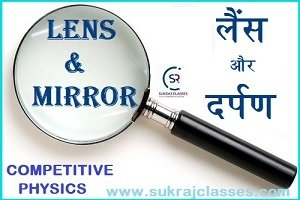# Lens and Mirror – लैंस और दर्पण – Competitive Physics

Physics topic- “Lens and Mirror – लैंस और दर्पण”, is important for all competitive exams like CET- Common eligibility Test,  SSC CGL, SSC CHSL, RRB NTPC, UPSC and other state civil services exams. In these exams, almost 4-5 questions are coming from Physics. Let’s start the topic:

## (लैंस और दर्पण)

Lens (लैंस):

A lens is a piece of transparent material that bound by two surfaces, of which one or both surfaces are spherical. Lens is shaped as which make cause light rays to bend in a specific way as they pass through it, whether this make converge or diverge to a specific point.

The material used could be a piece of glass or plastic, and the shape of the lens determines whether it causes light rays to converge or diverge.

Type of lenses (लैंस के प्रकार):

The classification of a lens depends on how the light rays bend when they pass through the lens. The two main types of lenses are:

There are two basic type of lenses:

1. Convex Lens (Converging)
2. Concave Lens (Diverging)

Convex lenses (उत्तल लेंस):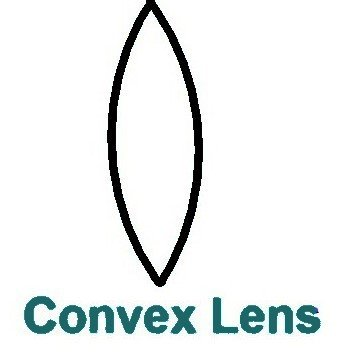A convex lens is a lens that converges rays of light that are traveling parallel to its principal axis. So, this is also called “converging lens”.

This lens is thick at the center but thin at the edges.”

Principal axis: An imaginary straight line passing through the two centres of curvature of a lens is called its principal axis.

Centers of curvature: – A lens, either a convex lens or a concave lens, has two spherical surfaces. Each of these surfaces forms a part of a sphere. The centres of these sphere are called ‘centres of curvature’ of the lens.

Use of convex lenses:

• It is used to correct Hypermetropia or long-sightedness.
• It is used in magnifying devices like microscopes, telescopes and camera lenses.

Concave lenses (अवतल लेंस):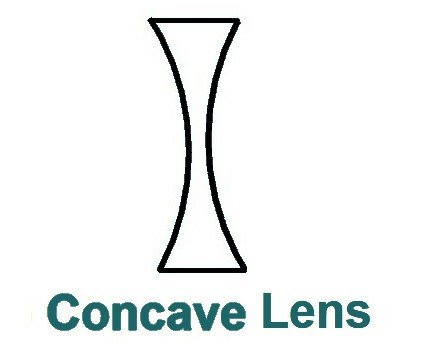The lens causes the parallel rays of light to diverge from a point. This is called a concave or diverging lens. This lens is thin at the center and thick at the edges.

Use of concave lens:

• Concave lens is used to correct myopia or nearsightedness.
• Concave lens is used in telescope and binoculars to magnify objects.
• Concave lens also used in flashlight.

Difference between the convex and concave lens: –

 Convex lens Concave lens Convex lens has positive power. So, it is also called positive lens. Concave lens has negative power. So, it is also called negative lens. It is converging lens. It is diverging lens. It forms real image. It forms virtual image. Its principal focus is real. Its principle focus is virtual. It is thick in middle. It is thick at corners. It is thin at corners. It is thin at middle.

Power of lens (लेंस की क्षमता): The degree of convergence or divergence of light rays achieved by a lens is expressed in terms of “Lens Power”.

• It is represented by “P”.
• The S.I. Unit of power of a lens: – Diopter (D)

Some important points of lenses:-

• If a lens immersed in water, its nature will be change.
• It means concave lens act as convex lens and convex lens act as concave lens.
• Shop bubbles act as concave lens inside the water which is opposite nature of the shop bubble.

Mirror (दर्पण):

A mirror is a reflective surface that light does not pass through, but bounces off and this produces either a real image or a virtual image.

• Mirror is polished with mercury vapors.
• Silver is very commonly used because it is the best reflector of visible light amongst common metals.
• When you place an object in front of a mirror, you see the same object in the mirror. The object is the source of the incident rays and the image is formed by the reflected rays.

The main two types of mirrors are:

• Plane Mirrors (समतल दर्पण)
• Spherical Mirrors (गोलीय दर्पण)

Plane Mirrors (समतल दर्पण): –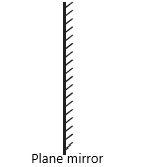• A plane mirror is a flat, smooth reflective surface.
• A plane mirror always forms a virtual image of the same shape and size as the object.

Some important points of plane mirrors:

• Image is formed double of mirror length, i.e. for a full image we need of a half-length mirror.
• If two simple mirror kept parallel, infinite (odd maximum) image form in between them.
• Simple mirrors are used in the decoration of the showrooms. Due to which size of showrooms appear larger than actual.

Spherical Mirrors (गोलीय दर्पण):

• A spherical mirror is a mirror that has a consistent curve and a constant radius of curvature.
• The images formed by a spherical mirror can either be real or virtual.
• Spherical mirrors are the mirrors having curved surfaces that are painted on one of the sides.

Spherical mirrors are two types:

• Concave Mirror (अवतल दर्पण)
• Convex Mirror (उत्तल दर्पण)

Concave Mirror (अवतल दर्पण):-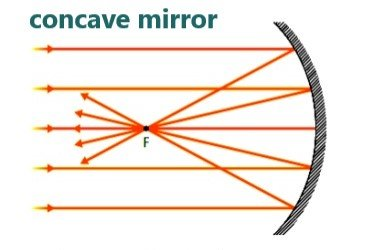Spherical mirrors in which inward surface is painted, is known as concave mirrors.

Light converges at a point when it strikes and reflects back from the reflecting surface of the concave mirror. Hence, it is also known as a converging mirror.

• In concave mirror image is formed larger than object.
• It is used in vanity box and in museum.
• It is used in headlight of vehicles.

Convex Mirror (उत्तल दर्पण):-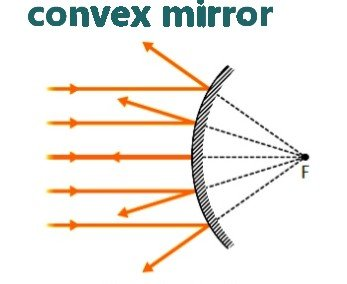• The spherical mirrors in which outward surface is painted, is known as convex mirrors.
• A convex mirror is sometimes referred to as a diverging mirror due to the fact that incident light originating from the same point and will reflect off the mirror surface and diverge.
• In convex mirror image formed smaller than object.
• convex mirror use as a rear view mirror in the vehicles because convex mirror always forms virtual, erect and diminished images irrespective of distance of the object. A convex mirror enables a driver to view large area of the traffic behind him.

Difference between Mirrors and Lens:

 Mirror Lens Mirrors reflect to light rays. Lens refract to light rays. A mirror will have only one focal point, which is in front of the mirror. A lens has two focal points each on either side A mirror is a glass surface with a silvery backing that produces an image through reflection. A lens is a transparent substance of glass or plastic, bound by two surfaces, whose at least one surface is curved. It can either be plane or curved It is usually curved The working principle of the mirror is the “law of reflection”. The working principle of the mirror is the “law of refraction”.

For More:

If you like and think that General Science (Physics) topic on “Lens and Mirror – लैंस और दर्पण” was helpful for you, Please comment us. Your comments/suggestions would be greatly appreciated. Thank you to be here. Regards – Team SukRaj Classes.